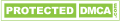# php if else statement

Programming language can contain the code of one line or many lines. To control the execution of all these code lines, use the if statement if the statement is of 3 types -

1 if statement

2 if else statement

3 if else if statement

## 1 if statement

If a condition is given in the if statement, the code is run if the condition is true and if the content given in is displayed in the web page, if the condition is false then the code is not run and the output in the web page is not displayed anything.

Syntax

if(expression){

//content

}

Example 1

File name : index.php



Output

 7 < 10

## 2 if else statement

If a condition is given in the if statement, the code is run if the condition is true and if the content given in it is displayed in the web page, if the condition is false then the content in the else is displayed in the web page.

Syntax

if(expression){

//content display if condition is true

}

else{

//content display if condition is false

}

Example 2

File name : index.php

 \$num2"; } ?>

Output

 17 > 10

## 3 if else if statement

If a condition is given in the if statement, if the condition is true then the statement given in if is displayed in the web page, if the condition is not true then interpreter checks all else if statement if it also has no condition true then in else The given code is run.

In this, you can use two or more else if statements.

Syntax

if(expression1){

//content display if expression1 is true

}

else if(expression2){

//content display if expression2 is true

}

else if(expression3){

//content display if expression3 is true

}

else{

//content display if expression is false

}

Example 3

File name : index.php

 \$num2"; } ?>

Output

 10 = 10

### Connect with us###### Email : contact@amcodestar.com